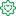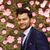Announcements
The way to achieve your own success is the willingness to help somebody else. Go for it!Former Employee

## Use Aggregation Functions!

On the discussion forum, I often see people posting questions around expressions that don’t work. When looking at the descriptions, I usually find that the reason is that the expressions lack aggregation functions. So, here is a suggestion...

Always use an aggregation function in your expression.

The reason is that a field reference in an expression always means an array of values. Which in turn means that you must enclose it in an aggregation function to make it collapse into one value:

OrderDate             An array of values

Max(OrderDate)     A single value

If you don't use an aggregation function, QlikView will use the Only() function. Hence, if the field reference returns several values, QlikView will interpret it as NULL, and the expression will not be evaluated the way you want it to.

Example 1: Use of the If() function:

If() functions are often used for conditional aggregations:

If( OrderDate >= vReferenceDate, Sum(Amount) )

At first glance, this expression may look correct: For dates after a reference date, the field Amount should be summed. Right?

Wrong.

OrderDate is a naked field reference: It does not have an aggregation function. Hence, it is an array, possibly with several values, and if so, evaluates to NULL. If you are lucky, there is only one date per dimensional value in your chart, and the expression will calculate fine. However, QlikView will probably not be able to calculate the expression for the subtotals in the chart, since there for those exists several dates.

A correct expression that always works should use a Min() or some other aggregation function in the first parameter of the If() function:

If( Min(OrderDate) >= vReferenceDate, Sum(Amount) )

Or, alternatively, the If() function should be put inside the Sum() function:

Sum( If(OrderDate >= vReferenceDate, Amount) )

In the first of the two expressions, the If() function will be evaluated once per dimensional value; in the second once per row in the raw data. The results are slightly different, but both return an answer, as opposed to the original expression. The picture below shows the difference between the expressions, using 2013-02-01 as reference date.Example 2: Sort by expression:

The expression used to sort the dimensional values in a chart is also an aggregation. Often you don’t think about this since you choose an expression that returns just one value per dimensional value, and then a naked field reference works fine.

But sometimes this still doesn't work…

For example, say that you want to show support cases in a CRM system. You create a chart with the support case as dimension and some measure as expression. Of course you want to sort the support cases chronologically, so you use "Sort by Expression" and as expression you choose

[Opening Date]

This will work in most cases. However, some CRM systems allow you to re-open a support case, hence assigning two opening dates to one single support case.  For these cases, the above expression will not work.

Instead, you should always ask yourself which function to use, should there be two values. The answer is usually Sum(), Avg(), Min() or Max(). In the above case, you should use

Min([Opening Date]) , or

Max([Opening Date])

depending on whether you want to use the first or last date.

Bottom line: Use aggregation functions, not just in your chart measures, but also in sort expressions, labels, show conditions, calculation conditions, text boxes, sheet names and searches.

HIC

Further reading related to this topic:

It’s all Aggregations

Aggregations and Function ClassesPartner - Contributor III

Situation, I have a table of values, A, B, and C.  I'm trying to calculate every result, where A = B * C.  I don't want to only calculate the Max or Min or Avg of B * C, rather, I want every instance of A calculated for every B and C.  I want it to work as if it was being calculated in a spreadsheet.  What aggregation function do I use for this situation?

466 ViewsContributor III

Hi @hic  wanted to understand the functioning of the expression for 2nd dimension value.

For second dimension value we have null value in 2nd expression but there is some value in 3rd expression of this dimension value.

If i visualize the functioning of expression i have few observation:

1. For 'Q1 2013' , suppose we have many values of OrderDate values, and the MIN(OrderDate) will give me the minimum value for 'Q1 2013' but could not understand how 3rd expression is giving value for this dimension value i.e. 'Q1 2013' as the 3rd expression is executing at the raw data level and if there is any OrderDate value which is less than the 'vRefrenceDate' variable for 'Q1 2013' dimension value , it implies there is OrderDAte value which is less than the vRefrenceDate then why it is not giving result for second expression.

Please try to explain me ass i am not able to visualize it.

225 Views
Subscribe by Topic